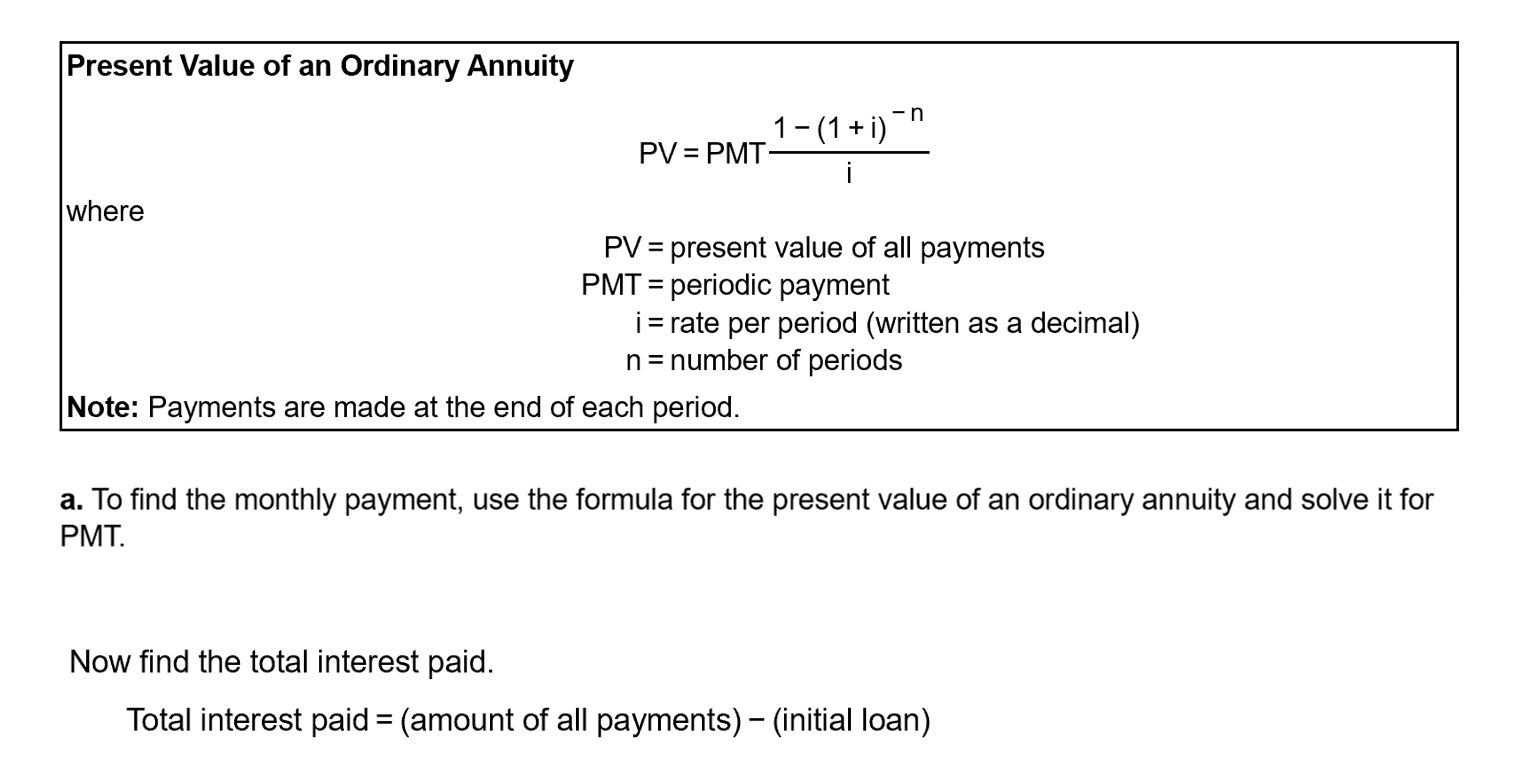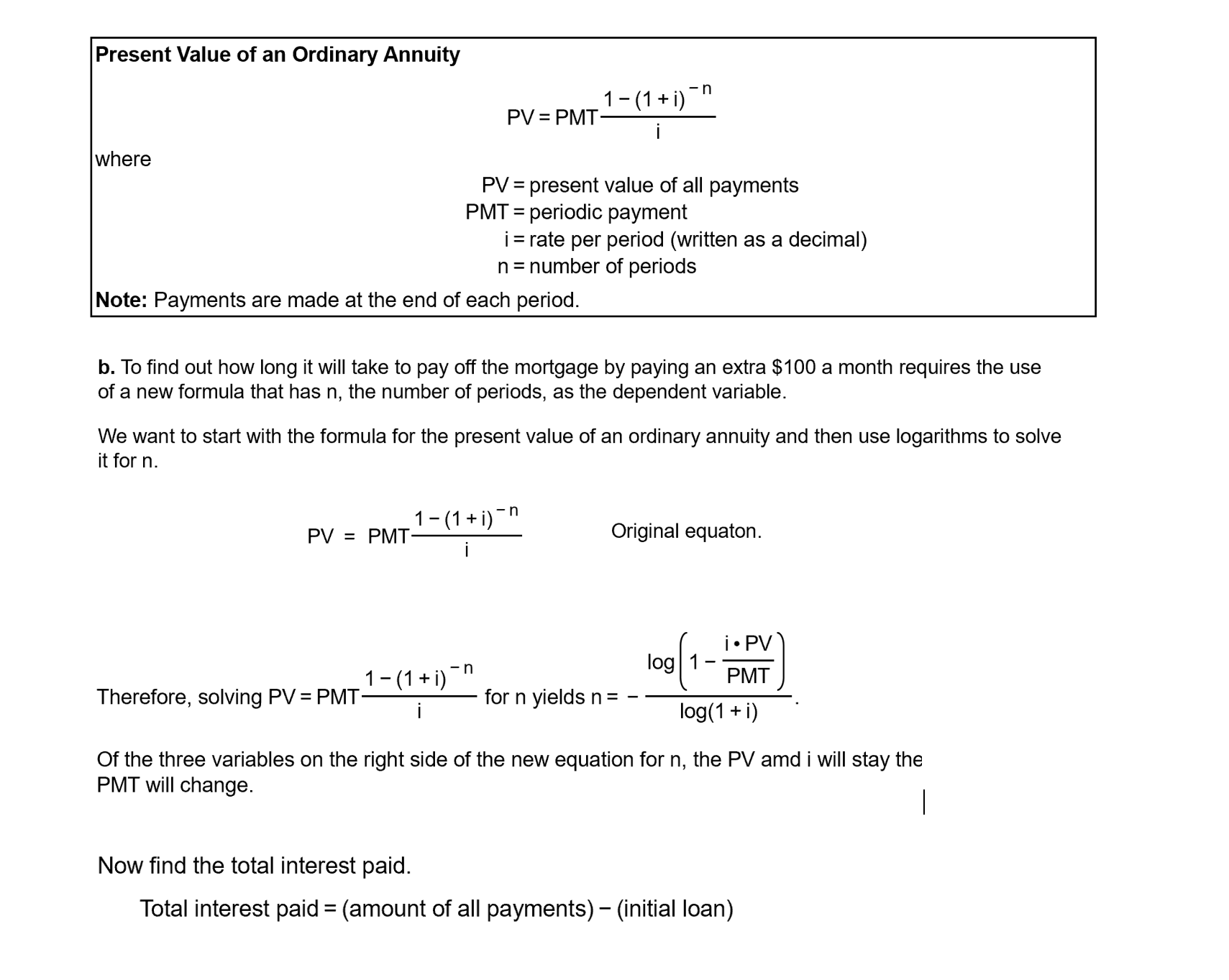# Present Value of an Ordinary Annuity 1- (1 + i) PV = PMT- i where PV = present value of all payments PMT = periodic payment i= rate per period (written as a decimal) n= number of periods %3D Note: Payments are made at the end of each period. a. To find the monthly payment, use the formula for the present value of an ordinary annuity and solve it for PMT. Now find the total interest paid. Total interest paid = (amount of all payments) - (initial loan) Present Value of an Ordinary Annuity -n 1-(1 + i) PV = PMT- where PV = present value of all payments PMT = periodic payment i= rate per period (written as a decimal) n= number of periods %3D %3D Note: Payments are made at the end of each period. b. To find out how long it will take to pay off the mortgage by paying an extra $100 a month requires the use of a new formula that has n, the number of periods, as the dependent variable. We want to start with the formula for the present value of an ordinary annuity and then use logarithms to solve it for n. 1-(1+ i) -n PV = PMT: Original equaton. i• PV log 1 1-(1+ i)" PMT Therefore, solving PV = PMT for n yields n= log(1 + i) Of the three variables on the right side of the new equation for n, the PV amd i will stay the PMT will change. Now find the total interest paid. Total interest paid = (amount of all payments) – (initial loan) Question A family has a$145,247​, 15-years mortgage at 5.7 % compounded monthly.
(A) Find the monthly payment and the total interest paid. (equations on picture - equations for problem A)
Monthly​ payment: $____ (Round to two decimal​ places.) Total interest​ paid:$____ (Round to two decimal​ places.)

(B) Suppose the family decides to add an extra​ $100 to its mortgage payment each month starting with the very first payment. How long will it take the family to pay off the​ mortgage? How much interest will the family​ save? (equations on picture - equations for problem B) Time: ____ years (Round to two decimal​ places.) Total interest​ paid:$____ (Round to two decimal​ places.)help_outlineImage TranscriptionclosePresent Value of an Ordinary Annuity 1- (1 + i) PV = PMT- i where PV = present value of all payments PMT = periodic payment i= rate per period (written as a decimal) n= number of periods %3D Note: Payments are made at the end of each period. a. To find the monthly payment, use the formula for the present value of an ordinary annuity and solve it for PMT. Now find the total interest paid. Total interest paid = (amount of all payments) - (initial loan) fullscreenhelp_outlineImage TranscriptionclosePresent Value of an Ordinary Annuity -n 1-(1 + i) PV = PMT- where PV = present value of all payments PMT = periodic payment i= rate per period (written as a decimal) n= number of periods %3D %3D Note: Payments are made at the end of each period. b. To find out how long it will take to pay off the mortgage by paying an extra \$100 a month requires the use of a new formula that has n, the number of periods, as the dependent variable. We want to start with the formula for the present value of an ordinary annuity and then use logarithms to solve it for n. 1-(1+ i) -n PV = PMT: Original equaton. i• PV log 1 1-(1+ i)" PMT Therefore, solving PV = PMT for n yields n= log(1 + i) Of the three variables on the right side of the new equation for n, the PV amd i will stay the PMT will change. Now find the total interest paid. Total interest paid = (amount of all payments) – (initial loan) fullscreen

## Expert Answer

### Want to see this answer and more?

Experts are waiting 24/7 to provide step-by-step solutions in as fast as 30 minutes!*

*Response times vary by subject and question complexity. Median response time is 34 minutes and may be longer for new subjects.
Tagged in
BusinessFinance

### Mortgages© 2021 bartleby. All Rights Reserved.